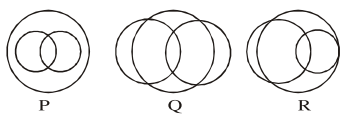# In a school,

Question:

In a school, there are three types of games to be played. Some of the students play two types of games, but none play all the three games. Which Venn diagrams can justify the above statement?1. $P$ and $Q$

2. $\mathrm{P}$ and $\mathrm{R}$

3. None of these

4. $Q$ and $R$

Correct Option: , 3

Solution:

$A \cap B \cap C$ is visible in all three venn diagram

Hence, Option (3)